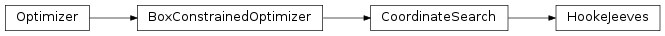# 4.5. `pyopus.optimizer.hj` — Box constrained Hooke-Jeeves optimizer¶Box constrained Hooke-Jeeves optimizer (PyOPUS subsystem name: HJOPT)

An extended version of coordinate search where so-called speculative steps are taken from time to time. These steps are hoped to speed up the search.

The convergence theory applied to coordinate search can also be applied to Hooke-Jeeves algorithm if the speculative step length factor is an integer.

The algorithm (unconstrained version) was first published in [hj].

 [hj] Hooke R., Jeeves T. A., Direct Search Solution of Numerical and Statistical Problems. Journal of the ACM (JACM), vol. 8, pp. 212-229, 1961.
class `pyopus.optimizer.hj.``HookeJeeves`(function, xlo=None, xhi=None, debug=0, fstop=None, maxiter=None, stepup=1.0, stepdn=0.5, step0=None, minstep=None, speculative=2.0)

Hooke-Jeeves optimizer class

speculative is the step length factor for the speculative step.

See the `CoordinateSearch` class for more information.

`check`()

Checks the optimization algorithm’s settings and raises an exception if something is wrong.

`reset`(x0)

Puts the optimizer in its initial state and sets the initial point to be the 1-dimensional array or list x0. The length of the array becomes the dimension of the optimization problem (`ndim` member). The shape of x0 must match that of xlo and xhi.

`run`()

Runs the optimization algorithm.

Example file hj.py in folder demo/optimizer/

```# Optimize Rosenbrock function with Hooke-Jeeves optimizer.

from pyopus.optimizer.hj import HookeJeeves
from pyopus.problems.mgh import Rosenbrock

if __name__=='__main__':
prob=Rosenbrock()
opt=HookeJeeves(prob.f, debug=1, maxiter=100000, step0=1e-1, minstep=1e-6)
opt.reset(prob.initial)
opt.run()

print("x=%s f=%e" % (str(opt.x), opt.f))
```